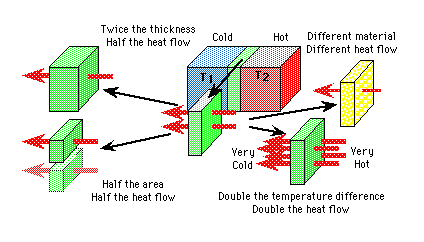# Hands-On-PhysicsHEAT & TEMPERATURE

## - Mathematics-

### Converting Between C and F

You often need to convert between the Celsius and Fahrenheit scales. Call c the temperature of something in Celsius and f the same temperature expressed as Fahrenheit. Then:
• f = 32 + 9 c / 5
• c = 5 (f - 32)/ 9

### Heat Flow Equation

Assume one object is at temperature T1, the other is at T2, and that T2 is hotter than T1. Focus your attention to the material between the objects. Call Q the heat flowing from T2 to T1 that must flow through this material. Several factors influence how much heat will flow through this material:
• Thickness.
The thickness, d, of the contact material will influence the heat flow. If you keep everything else constant, but increase the thickness, it will be more difficult for heat to flow. If you double the thickness, only half the heat will flow. If you cut the thickness in half, the heat flow will double.
• Area.
The area, A, of the material in contact with the two objects will influence the amount of heat flow. If you keep everything else constant, but increase this area, the heat flow will double. If you cut the area in half, the heat flow will be halved, also.
• Temperature difference.
The temperature difference, T2 - T1 influences the amount of heat flow. The greater the temperature difference, the more heat will flow. Double this difference and the heat flow will double, too.
• Material.
Of course the kind of material has a huge impact on the amount of heat flow. Some materials conduct heat very well and others - insulators - do not. The specific conductivity, K, is a measure of the ability of any material to let heat flow through it. The larger K, the more heat will flow, if all other variables are kept constant. Double the value of K and the heat flow will double.

•Figure P8
Heat Flow

All these factors can be summarized in one equation:

Q = KA(T2 - T1)/d

Q is the heat flow through the conductor from substance-2 to substance-1. K is the specific conductivity of the conductor, A is the cross-sectional area of the conductor in contact with the substances. T2 - T1 is the temperature difference across the conductor. d is the thickness of the conductor.

TOP
Previous Page || Up a Level || Index || Next Page